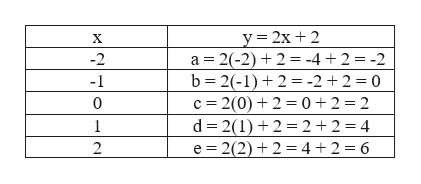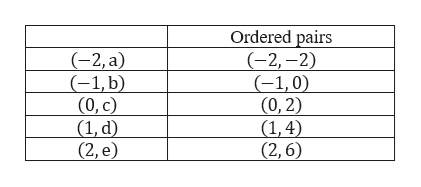# Given the table, find 5 solutions to the equation.xy=2x+2-2a-1b0c1d2eEnter as an ordered pair.(−2,a)= (−1,b)= (0,c)=(1,d)= (2,e)= Plot the 5 solutions found above.Clear All Draw: Dot LicensePoints possible: 1Unlimited attempts.

Question
3 views
Given the table, find 5 solutions to the equation.

x y=2x+2
-2 a
-1 b
0 c
1 d
2 e

Enter as an ordered pair.
(−2,a)=
(−1,b)=
(0,c)=
(1,d)=
(2,e)=

Plot the 5 solutions found above.

Clear All Draw: Dot

Unlimited attempts.
check_circle

Step 1

Given the table, to find 5 solutions to the equation.

Step 2

Substitute the value of x in the equation to find y.help_outlineImage Transcriptionclosey=2x + 2 a 2(-2) 2= -4 +2 = -2 b 2(-1)2= -2 +2 = 0 X -2 -1 0 c 2(0)2 0 2 2 d 2(1)2 2 2 = 4 e 2(2)2= 4+ 2 = 6 1 2 fullscreen
Step 3

Now, to enter them as ...help_outlineImage TranscriptioncloseOrdered pairs (-2,-2) (-1,0) (0,2) (1,4) (2,6) (-2, а) (-1, b) (0, с) (1, d) (2, e) fullscreen

### Want to see the full answer?

See Solution

#### Want to see this answer and more?

Solutions are written by subject experts who are available 24/7. Questions are typically answered within 1 hour.*

See Solution
*Response times may vary by subject and question.
Tagged in

### Equations and In-equations